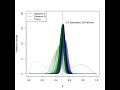# Posts Tagged ‘ Bernoulli ’

## An update on visualizing Bayesian updating

August 17, 2012
ByA while ago I wrote this post with some R code to visualize the updating of a beta distribution as the outcome of Bernoulli trials are observed. The code provided a single plot of this process, with all the curves overlayed on top of one another. Then John Myles White (co-author of Machine Learning for

## Visualizing Bayesian Updating

September 10, 2011
ByOne of the most straightforward examples of how we use Bayes to update our beliefs as we acquire more information can be seen with a simple Bernoulli process. That is, a process which has only two  possible outcomes. Probably the most commonly thought of example is that of a coin toss. The outcome of tossing

## Another Bernoulli factory

February 13, 2011
ByThe paper “Exact sampling for intractable probability distributions via a Bernoulli factory” by James Flegal and Radu Herbei got posted on arXiv without me noticing, presumably because it came out just between Larry Brown’s conference in Philadelphia and my skiing vacations! I became aware of it only yesterday and find it quite interesting in that

## Will I ever be a bayesian statistician ? (part 1)

January 20, 2011
ByLast week, during the workshop on Statistical Methods for Meteorology and Climate Change (here), I discovered how powerful bayesian techniques could be, and that there were more and more bayesian statisticians. So, if I was to fully understand app...

## A probability exercise on the Bernoulli distribution

July 18, 2009
ByWhat is the probability, flipping a coin 8 times, to obtain the sequence HHTTTHTT? (H = head; T= tail)The theory teaches us that to solve this question, we can simply use the following formula:f(x)=P(X=x)=B(n,p)=\begin{pmatrix}n\\ x \end{pmatrix} \cd...